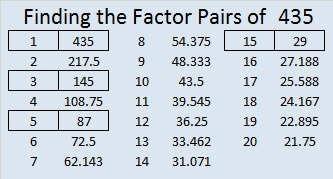# 435 is a Hexagonal Number

It isn’t too hard to visualize the first three hexagonal numbers and count 1, 6, or 15 dots. Any more than that boggles my mind so I made a less mind-boggling chart with the first fifteen hexagonal numbers. In the picture on the chart you can see the first dot that makes the first hexagonal number and several other hexagonal numbers embedded in the the seventh hexagonal number that has 91 dots. Counting all those dots can be quite tedious!The nth hexagonal number = n(2n – 1). That means 435 (the 15th hexagonal number) can be written 435 = 15 (2 x 15 – 1) = 15 x 29.

435 is also the 29th triangular number because 435 = 29 (29 + 1)/2 = 29 x 15. (All hexagonal numbers are also triangular numbers.)  Being the 29th triangular number means 1 + 2 + 3 + 4 + . . . + 26 + 27 + 28 + 29 = 435

The other factors of 435 are listed below the puzzle.Print the puzzles or type the factors on this excel file: 12 Factors 2015-03-23

• 435 is a composite number.
• Prime factorization: 435 = 3 x 5 x 29
• The exponents in the prime factorization are 1, 1, and 1. Adding one to each and multiplying we get (1 + 1)(1 + 1)(1 + 1) = 2 x 2 x 2 = 8. Therefore 435 has exactly 8 factors.
• Factors of 435: 1, 3, 5, 15, 29, 87, 145, 435
• Factor pairs: 435 = 1 x 435, 3 x 145, 5 x 87, or 15 x 29
• 435 has no square factors that allow its square root to be simplified. √435 ≈ 20.8567## One thought on “435 is a Hexagonal Number”

This site uses Akismet to reduce spam. Learn how your comment data is processed.